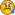Hello Guest

# Application crash when using VBO

• 1 Replies
• 4335 Views####julien.1486

•• 8##### Application crash when using VBO
« on: March 03, 2009, 19:27:30 »
Hi !

I try to use the VBOs in order to render a model but my application crash when I call glDrawRangeElements.

I have a "ObjectGL" class which can contain several "Materials" objects (which are polygons with specific materials datas).

Firstly, I generate the VBO id for each Materials (they are stored in the ArrayList "mats") :
Code: [Select]
`public void genVBOS() throws IOException{ if(GLContext.getCapabilities().GL_ARB_vertex_buffer_object){ for (int i = 0; i < mats.size(); i+=2) { //One for datas, one for indices IntBuffer vbo = BufferUtils.createIntBuffer(2); ARBVertexBufferObject.glGenBuffersARB(vbo); ARBVertexBufferObject.glBindBufferARB(ARBVertexBufferObject.GL_ARRAY_BUFFER_ARB, vbo.get(i)); ARBVertexBufferObject.glBufferDataARB(ARBVertexBufferObject.GL_ARRAY_BUFFER_ARB, vvtvn, ARBVertexBufferObject.GL_STATIC_DRAW_ARB); //vvtvn is an interleaved array of vertices, tex coords and normals ARBVertexBufferObject.glBindBufferARB(ARBVertexBufferObject.GL_ELEMENT_ARRAY_BUFFER_ARB, vbo.get(i+1)); ARBVertexBufferObject.glBufferDataARB(ARBVertexBufferObject.GL_ELEMENT_ARRAY_BUFFER_ARB, mats.get(i).getIndices(), ARBVertexBufferObject.GL_ELEMENT_ARRAY_BUFFER_ARB); mats.get(i).setVbo(vbo); } }`
And after I call the ObjectGL.draw function :

Code: [Select]
`public void draw(){ for (int i = 0; i < mats.size(); i++) { int stride = (3+2+3)*4;                       // Order : Vertices, Tex Coords, Normals ARBVertexBufferObject.glBindBufferARB(ARBVertexBufferObject.GL_ARRAY_BUFFER_ARB, mats.get(i).getVbo().get(0));    //The first ID contain the datas GL11.glEnableClientState(GL11.GL_VERTEX_ARRAY); int offset = 0; GL11.glVertexPointer(3, GL11.GL_FLOAT, stride, offset); if(normals){ GL11.glEnableClientState(GL11.GL_NORMAL_ARRAY); offset = 2 * 4; GL11.glTexCoordPointer(2, GL11.GL_FLOAT, stride, offset); } if(text){ GL11.glEnableClientState(GL11.GL_TEXTURE_COORD_ARRAY); offset = (3 + 2) * 4; GL11.glNormalPointer(GL11.GL_FLOAT, stride, offset); } ARBVertexBufferObject.glBindBufferARB(ARBVertexBufferObject.GL_ARRAY_BUFFER_ARB, mats.get(i).getVbo().get(1)); GL12.glDrawRangeElements(GL11.GL_TRIANGLES, 0, mats.get(i).getIndices().capacity(), mats.get(i).getIndices().capacity(), GL11.GL_UNSIGNED_INT, 0);    //At this point the application crashes } }`
I read some tutorials (including LWJGL documentation) about VBO but I guess something is wrong in my codeDo you have any idea ?
Thanks for your help##### Re: Application crash when using VBO
« Reply #1 on: March 07, 2009, 15:21:00 »
Hi,

it seems that you are running out of index range in genVBOS() when doing
Quote
ARBVertexBufferObject.glBindBufferARB(ARBVertexBufferObject.GL_ARRAY_BUFFER_ARB, vbo.get(i));
and
Quote
ARBVertexBufferObject.glBindBufferARB(ARBVertexBufferObject.GL_ELEMENT_ARRAY_BUFFER_ARB, vbo.get(i+1));
because the counter i is not guaranteed to be always in [0, 1], which is required, because the vbo names you are allocating are only 2 in size.

I guess you might use 0 as index for the first vbo.get() and 1 for the second one.